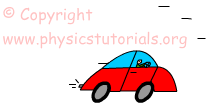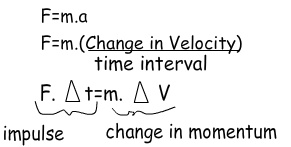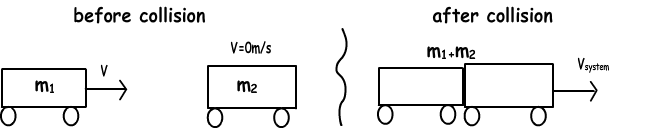## Impulse Momentum Cheatsheet

Impulse Momentum Cheat Sheet

Momentum

Momentum is a physical concept that is defined as “moving body”. In other words for talking about momentum we must have moving object, it must have both mass and velocity. Let me formulize what we said;

Momentum=Mass X Velocity=m.PWhere p is the momentum and m is the mass

Unit of the momentum is kg.m/s as you can guess from the equation.

Impulse

If you apply a force on an object 1 s then you see small change in the momentum. However, if you apply force on an object long period of time then you see the amount of change in momentum is bigger than the first situation. In summary, I try to say that impulse is the multiplication of applied force and time interval it applied. Impulse is also a vector quantity having both magnitude and direction. It has the same direction with applied net force.

Impulse=Force.Time Interval

Impulse and momentum are directly related to each other. Let’s find this relation now.Impulse=Change in Momentum

Conservation of Momentum

If there is no force applied on the system then momentum of the system is conserved in magnitude and direction.

Pinitial=Pfinal

m1.V1=m2.V2

Collisions

Momentum is conserved in all collisions. If the objects collide and momentum and kinetic energy of the objects are conserved than we call this collision “elastic collision”. On the other hand if the momentum of the object is conserved but kinetic energy is not conserved than we call this type of collision “inelastic collision”.To sum up, we can say that, momentum of the system is conserved in both elastic and inelastic collisions however; kinetic energy is conserved only in the elastic collisions.

Impulse Momentum Exams and Solutions

Author: